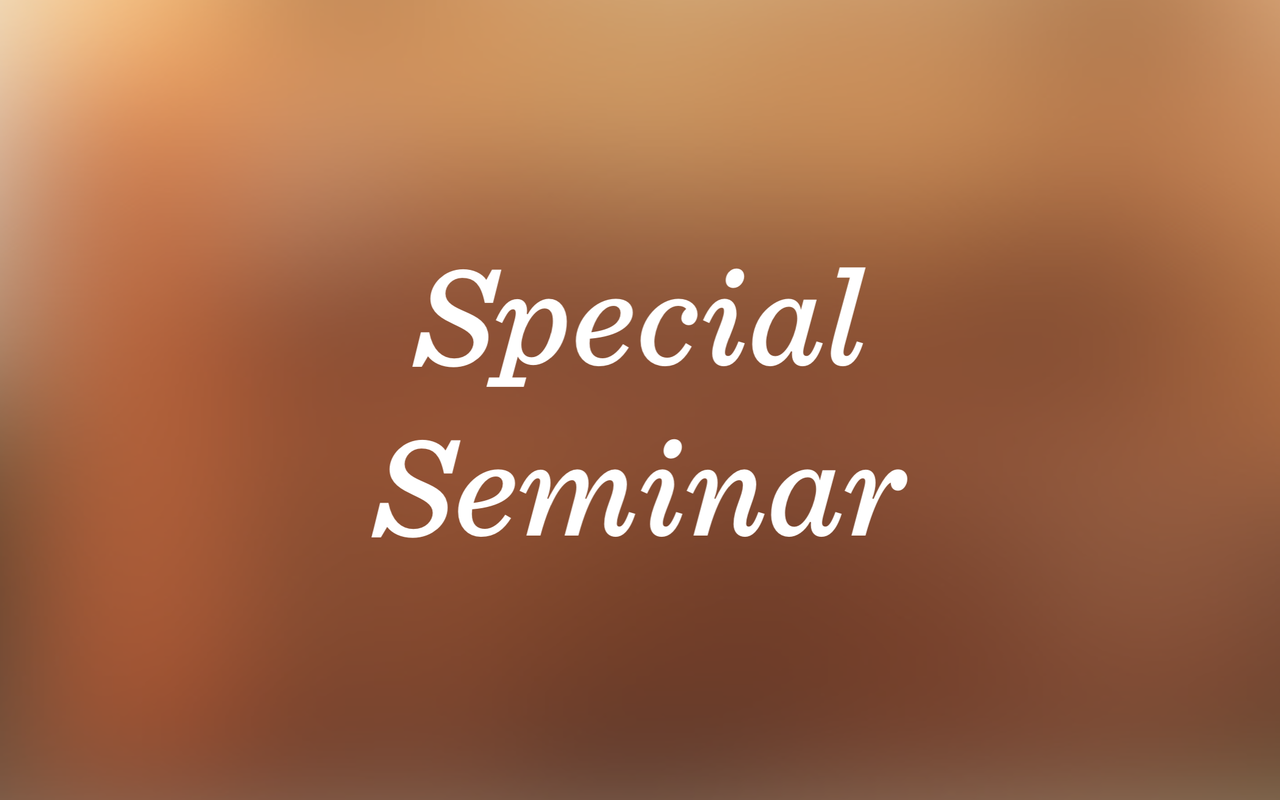# Special Seminar: Diptarka Chakraborty "Edit Distance: Constant-factor approximation, Embedding and Streaming"

This talk is arranged at the Department of Computer Science and it's open to everyone free-of-charge. The talk will take place at 09:00 (sharp!) in hall AS1, TUAS building.### Edit Distance: Constant-factor approximation, Embedding and Streaming

Diptarka Chakraborty

Abstract:

Edit distance is a measure of similarity of two strings based on the minimum number of character insertions, deletions, and substitutions required to transform one string into the other. This distance measure finds applications in fields such as computational biology, pattern recognition, text processing, and information retrieval. The edit distance can be computed exactly using a dynamic programming algorithm that runs in quadratic time, which is also known to be almost optimal under SETH assumption [Backurs, Indyk 2015]. Andoni, Krauthgamer and Onak (2010) gave a nearly linear time (randomized) algorithm that approximates edit distance within poly-log(n) approximation factor. In this talk we discuss a randomized algorithm with running time $\tilde{O}(n^{2-2/7})$ that approximates the edit distance within a constant factor. In the second half of this talk we discuss about a randomized embedding of the edit distance into the Hamming metric. We show a randomized embedding with quadratic distortion. More specifically, for any x,y satisfying that their edit distance equals k, the Hamming distance between the embedding of x and y is O(k^2) with high probability. This is the first randomized embedding having distortion factor independent of the length of the strings. Moreover, the embedding output size is linear in the input size and the embedding can be implemented in one-pass streaming model. Our embedding algorithm has several applications in document exchange, nearest neighbor search etc. and is also basis of a sketch based streaming algorithm for computing edit distance.

Bio:

I am currently a post-doctoral fellow at Weizmann Institute of Science, Israel. I am interested in theoretical computer science; more specifically small space computations, streaming algorithms, string matching algorithms (problems related to edit distance), graph algorithms and data structures. I did my PhD from Department of Computer Science & Engineering, Indian Institute of Technology, Kanpur. After that I spent two years at Charles University, Prague as a post-doctoral fellow and then in September 2018 I moved to Weizmann.

• Julkaistu:
• Päivitetty:
Jaa
URL kopioitu